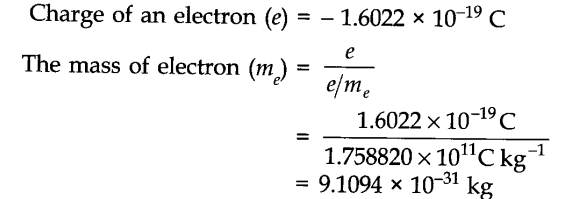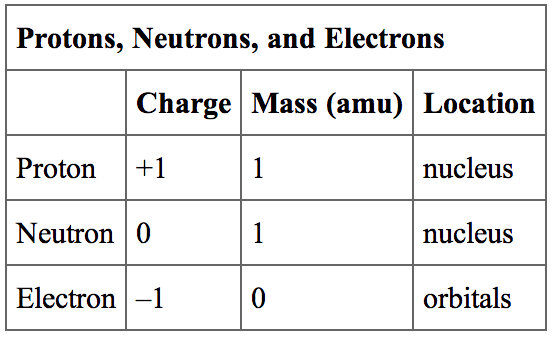# How To Calculate Electron Mass and Charge

The mass of the electron is also called the mass of a stationary electron. Because of its relation to the Avogadro constant, it is the fundamental constant of physics and is also very important in chemistry. Since it determines the number of observed effects in atomic physics so potentially there are many ways to determine its mass with an experiment.Earlier, the mass of the electron was determined directly by combining two measurements. Arthur Schuster in 1890 first estimated the mass-to-charge ratio of the electron by measuring the deflection of “cathode rays” by a known magnetic field in a cathode ray tube. It was before it was even known what cathode rays were. Seven years later J. J. Thomson showed that cathode rays consist of streams of particles and they are called electrons.

### Electron Mass Number

Mass of an Electron is calculated by 9.10938356 × 10-31 kilograms

### Electron Charge to Mass Ratio

eme = – 1.758820024(11)×1011 C/kg is the electron charge to mass ratio.### Proton Neutron Electron Mass

• Neutron Mass is 1.0086654 a.m.u. or 1.6749 x 10-27 kg.
• Electron Mass is 0.000548597 a.m.u. or 9.1 x 10-31 kg.
• Proton Mass is 1.0072766 a.m.u. or 1.6726 x 10-27 kg.

### Proton and Electron Mass

1.0072766 a.m.u. or 1.6726 x 10-27 kg is the mass of Proton and the mass of electron is 0.000548597 a.m.u. or 9.1 x 10-31 kg.

### Mass of Electron in Grams

9.1094e-28 is the mass of electron in Grams.